# COS 426 Assignment #2:Mesh Processing

## Data Structure Description

My data structure setup for this program was as follows:
• I started with an STL vector list of vertices, a vector list of edges, and a vector list of faces.
• Each vertex has a position, a normal, a texture coordinate (not actually used but in original implementation), and an STL Set of neighboring faces. The reason I chose to use a set to store neighboring faces was so that I could easily find the intersection between the sets of two adjacent vertices in an edge, and hence find the faces that come out of an edge. It also allowed me to easily get a vertex's neighbors
• Each face has a vector of 3 vertices, a vector list of edges(which ends up being 3 after the construction is over), and a plane. The plane usually isn't calculated unless it's actually needed
• Each edge has a pointer to its two vertices, and a pointer to the vertex at its midpoint. The reason I added the pointer to the vertex midpoint was because it was so convenient to have for functions like Fractalize() and Subdivide(). In many functions, it's not used, so it's simply null. But when I begin to split edges, it's very convenient to have a pointer that allows me to easily access the center.

## Transformations and Warps

### Random Noise

`void R3Mesh::RandomNoise(double noise);`
Adds noise of a random amount and direction to the position of every vertex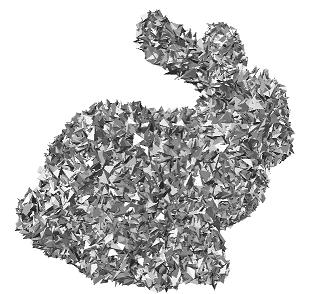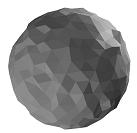meshpro bunny.off bunnynoise.off -noise 0.01 meshpro sphere.off spherenoise.off -noise 0.1

### Fun

`void R3Mesh::Fun(double omega, double factor);`
Do a sine bulge along the z-axis, using the x-axis as the argument to the sine, with frequency omega and amplitude factor.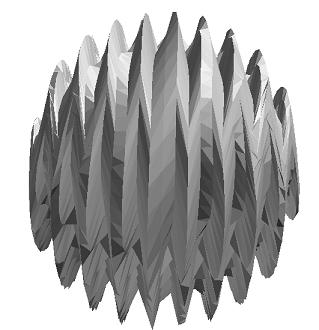meshpro sphere.off spherehighres.off -subdivide meshpro spherehighres.off spherefun.off -fun 30 0.2

### Scale

`void R3Mesh::Scale(double sx, double sy, double sz);`
Scale the mesh by increasing the distance from every vertex to the origin by a factor given for each dimension (sx, sy, sz).
 Original: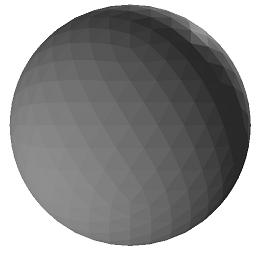Scaled: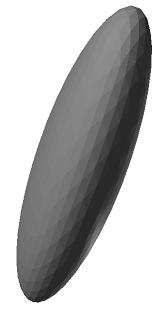meshpro sphere.off ellipse.off -scale 0.5 1 2

### Rotate

`void R3Mesh::Rotate(double angle, const R3Line& axis);`
Rotate the mesh counter-clockwise by an angle (in radians) around a line specified by a point (x, y, z) and a direction vector (dx, dy, dz).
No screenshot provided, since this one is self-explanatory and not-interesting (and hard to show with a screenshot).
Sample Execution:
meshpro input.off output.off -rotate 1 1 0 0 0 1
Rotates the mesh about the z-axis placed at (x=1, y=1)

### Inflate

`void R3Mesh::Inflate(double offset);`
Move every vertex by a given offset along its normal direction (this relies first on the successful execution of the compute vertex normals routine). This is as if every point were moved along the gradient of the surface (like moving out to an adjacent level set of an implicit suface).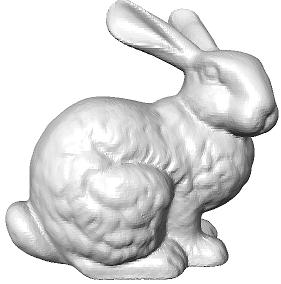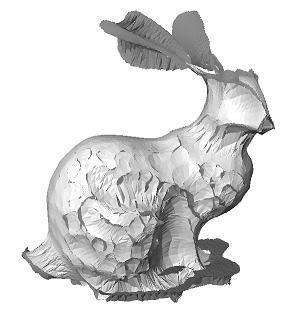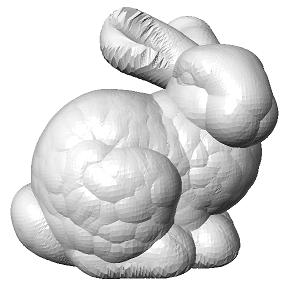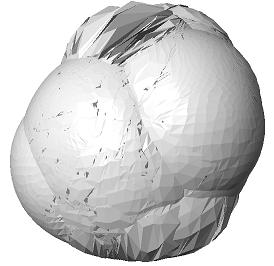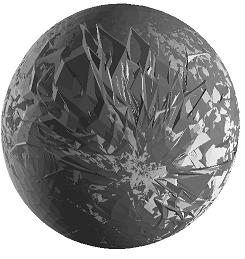Original meshpro bunny.off bunny-0.01.off -inflate -0.01(This one is technically "deflating" since the parameter is negative) meshpro bunny.off bunny0.01.off -inflate 0.01 meshpro bunny.off bunny0.1.off -inflate 0.1 meshpro bunny.off bunny1.off -inflate 1

### Deform According to Point Correspondences

`void R3Mesh::Deform(R3Point *source_points, R3Point *target_points, int npoints, double t);`
Deform according to point correspondences: Warp the mesh by a deformation defined by point correspondences provided in an ascii file with six numbers on each line: x y z x' y' z'. The position of every vertex in the output mesh should be offset by a Gaussian weighted average of the offsets implied by the point correspondences (x,y,z) -> (x',y',z'). The t parameter should control the "amount" of offset, so that t = 0 gives the original mesh, t = 1 gives the deformed mesh, and other t values interpolate or extrapolate. I chose to use the distance between the points in each correspondence as the width of the Gaussian.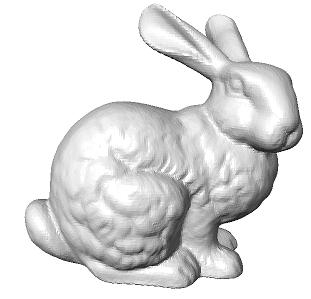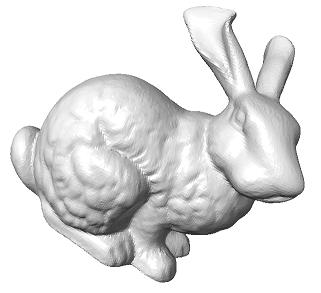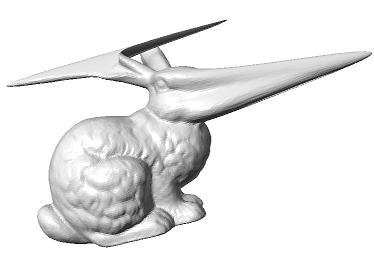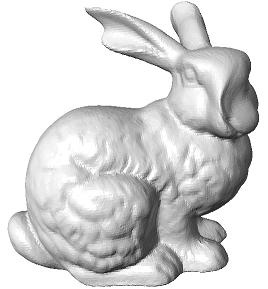Original meshpro bunny.off bunnydeform1.off -deform corr.txt 1 meshpro bunny.off bunnydeform10.off -deform corr.txt 10 meshpro bunny.off bunnydeform-1.off -deform corr.txt -1

## Filtering Operations

### Smooth

`void R3Mesh::Smooth(double sigma)`
Smooth the mesh by moving every vertex to a position determined by a weighted average of its immediate neighbors (wiht weights determined by a Gaussian function of distance in R3)

Use the equation G(dx,dy,dz, sigma) = e ^ (-(dx^2 + dy^2 + dz^2) / sigma^2), where dx, dy, and dz are the distance of a neighbor point from a central point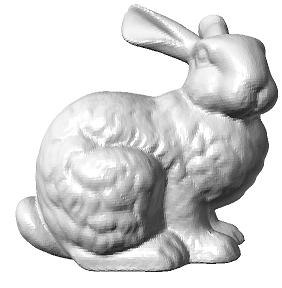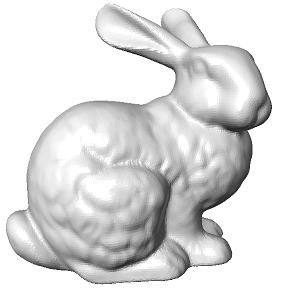meshpro bunny.off bunnysmooth.off -smooth 5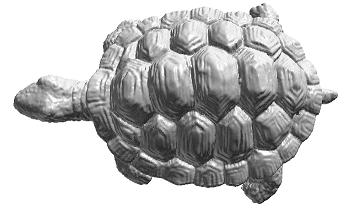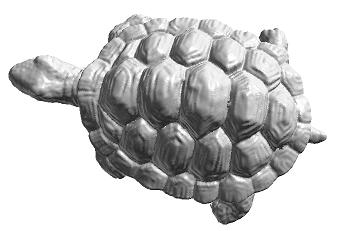meshpro turtle.off turtlesmooth.off -smooth 500

### Sharpen

`void R3Mesh::Sharpen(double sigma)`
Accentuate details in the mesh by moving every vertex away from the position determined by a weighted average of its neighbors (with weights determined by a Gaussian function).
If S is the smoothed position, P is the original position, P� = P + t*(P-S)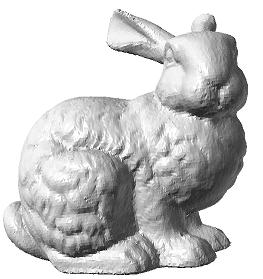meshpro bunny.off bunnysharp.off -sharpen 5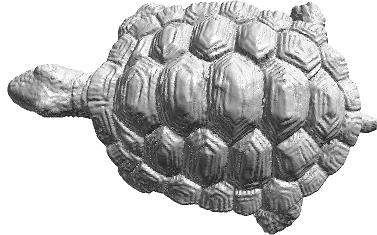meshpro turtle.off turtlesharp.off -sharpen 500

## Remeshing Operations

### Subdivide Faces

`void R3Mesh::Subdivide(void);`
Replace every triangle by four new ones. Create a new vertex at the midpoint of every edge, create new triangles between the vertices, and remove the original triangles.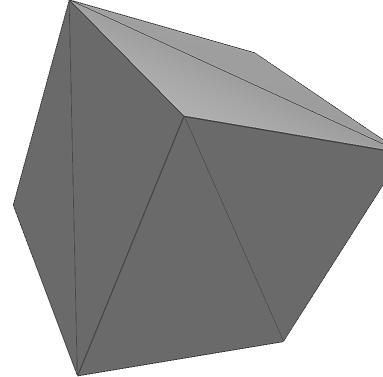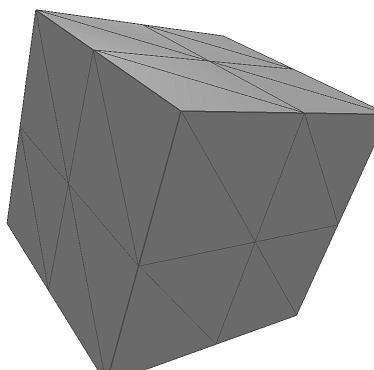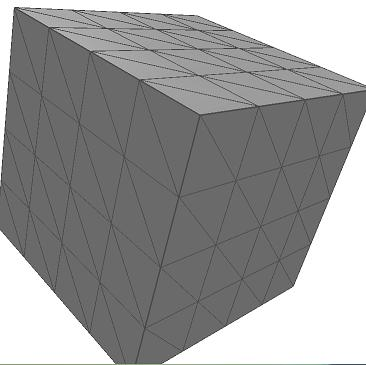Original meshpro cube.off cubediv1.off -subdivide meshpro cubediv1.off cubediv2.off -subdivide

## Analysis

### Compute Normals

`void R3Mesh::ComputeVertexNormals(void);`
Compute the surface normal at every vertex by taking a weighted average of the normals for the attached faces, where the weights are determined by the areas of the faces (like estimating the gradient of a level surface at discrete points). I take advantage of the fact that every vertex in my data structure points to its neighboring faces.
NOTE: No screenshot or mesh available; this operation is used in the inflate operation.

## Geometry Operations

### Surface of Revolution

```void R3Mesh::SurfaceOfRevolution(const R3Mesh& profile_curve,
const R3Line& axis_of_revolution, double rotation_angle_step);```
Take the cross sectional area described in profile_curve and rotate it by discrete amounts equal to rotation_angle_step around the line axis_of_revolution until it has rotated by 2PI. Join up each adjacent rotation of the cross section with faces. I relied heavily on my Rotation Routine here.

Execution:
meshpro int.off out.off -revolution file:profile real:px real:py real:pz real:vx real:vy real:vz real:angle_step
Where profile is a mesh that's only vertices (no faces) describing the cross-sectional area
The axis is (px, py, pz) * t * (vx, vy, vz) for a real number t
angle_step is the discrete rotation between adjacent faces
NOTE: The mesh "in.off" is ignored, but something needs to provided since I didn't get a chance to modify meshpro.cpp enough to deal with just an output mesh.

For this example, I used the cross-section provided in curv.off, which is the implicit curve defined by x = cos(a)+2; y = 0; z = sin(a) (a = [1/2*PI, 3/2*PI])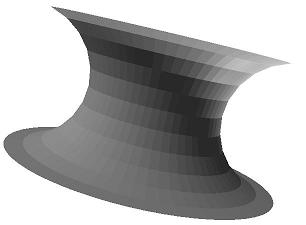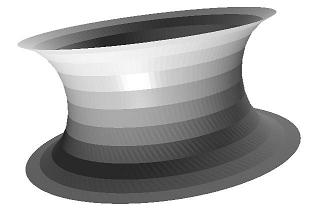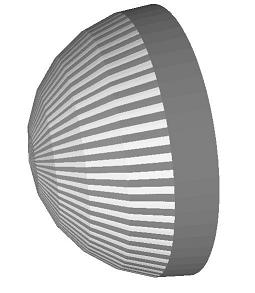meshpro bunny.off revolution1.off -revolution curv.off 0 0 0 0 0 1 0.1Rotate around the z-axis, using an angle step of 0.1 radians meshpro bunny.off revolution2.off -revolution curv.off 0 1 0 0 0 1 0.05Rotate around an offset of the z-axis this time, and use a smaller quantization for the angle (0.05 radians). Note how it's wider this time because of the placement of the rotation axis. meshpro bunny.off revolution3.off -revolution curv.off 0 0 0 1 0 0 0.1Rotate around the x-axis with a quantization of 0.1 radians

### Surface Sweep

```void R3Mesh::SurfaceSweep(const R3Mesh& crosssection_polygon, const R3Mesh& centerline_curve);
```
Take a cross-sectional area, provided in a mesh crosssection_polygon, and move it along the curve specified by another mesh, centerline_curve, to sweep out a surface from that area. In order for this to work and to have the right volume, the cross-sectional area needs to be rotated each time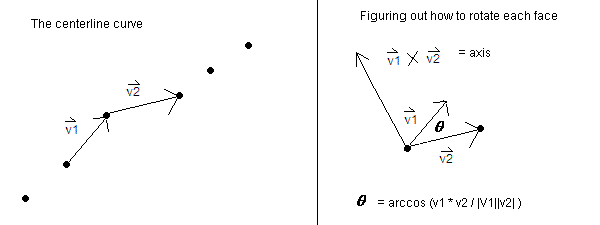In order to figure out how to rotate the cross-sectional area in each successive application of the sweep, I had to keep track of vectors that represented changes between points in the centerline curve. Taking the cross product of two of these successive vectors (v1 X v2, as shown in the picture above), gives the axis of rotation. Then, the angle between them gives the amount by which to rotate about that axis (I used the dot product to figure out theta).

I used the same cross-sectional area as in the surface of revolution, except I moved it across a line this time instead of rotating about an axis
NOTE: Once again, providing "bunny.off" as the input mesh is garbage, because the input mesh is simply ignored (the mesh is constructed from other paramters; namely, the cross-section area and the curve along which that area moves)
NOTE ALSO: The cross-sectional area is in curv.off, and the sweep line is in profile.off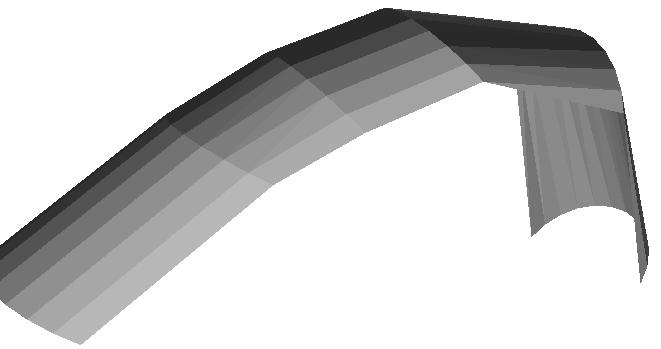meshpro bunny.off sweep.off -sweep curv.off profile.off

### Fractalize

`void R3Mesh::Fractalize(int nlevels);`
Create fractal detail by recursively subdividing every triangle of an input mesh into four (as in the Subdivide feature) and moving every new vertex by a random offset vector (as in the Random Noise feature) with magnitude proportional to the original edge length.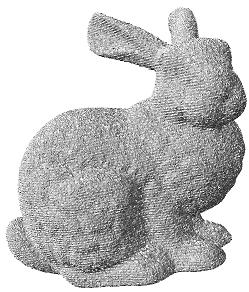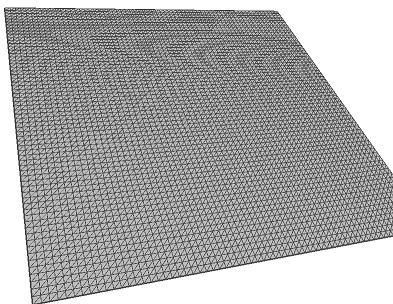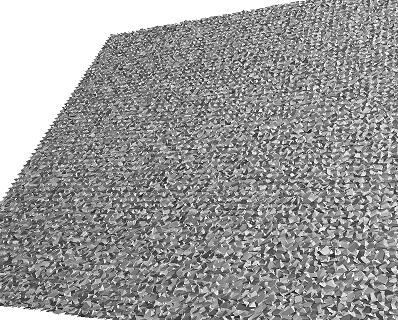meshpro bunny.off bunnyfrac.off -fractalize Original planar surface (after applying subdivide many times to one square to make the file fractalize.off) meshpro fractalize.off fractalize2.off -fractalize

## Topological Fixup

### Fill Holes

`void R3Mesh::FixHoles(void);`

Create triangles covering the holes of a mesh by connecting vertices on the boundary of every hole. Find holes by detecting a loop of edges that only have one face on them.
I created the following algorithm to do this:
1. Loop through all of the edges until an edge with only one neighboring face is found
2. Create an STL vector object that holds vertices, and add the first two vertices of that edge. Call them v1 and v2.
3. Get a list of all of the neighboring vertices of v2. If any of the edges between v2 and its neighbors have only one face attached, then add the neighbor to the set, and repeat the process for that vertex (recurse out)
4. If at any point in time, a neighbor vertex of the last vertex added is equal to the first vertex, that means the loop has been completed. Create a new vertex that's a weighted average of the loop vertices, and create faces between all of the loop vertices and that new center vertex
5. The recursion process will terminate when a hole has been filled, and the entire process will finished when all edges are checked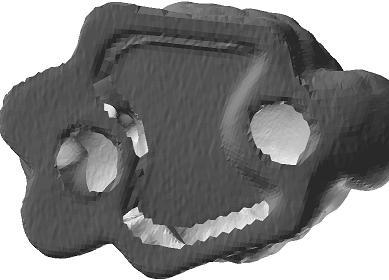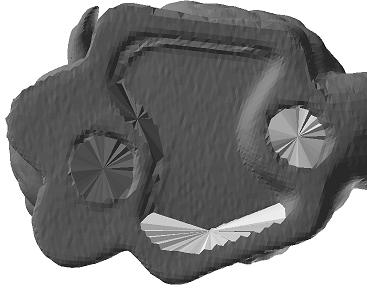meshpro bunny.off bunnyfix.off -fixholes

The surface of revolution I made has gaps at the top and the bottom. I used this algorithm to close that surface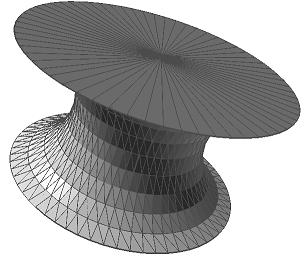meshpro revolution1.off revolutionfix.off -fixholes

## Miscellaneous Operations

### Creat a Mesh from an Image

`int R3Mesh::ReadImage(const char *filename)`

Create a mesh from a 2D image, with the x and y axes representing the pixel position in the image, and the z axis representing luminance of each pixel.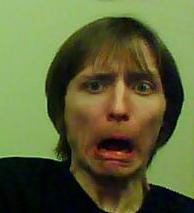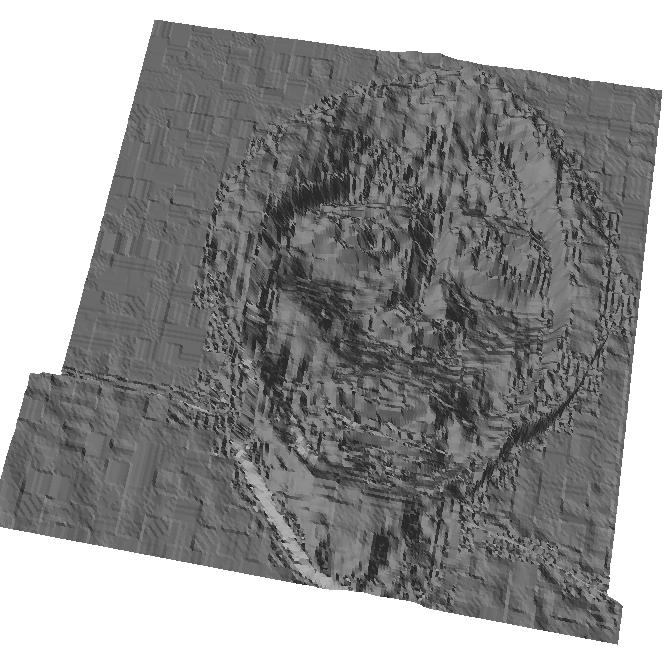meshpro bunny.off chris.off -image chris.jpg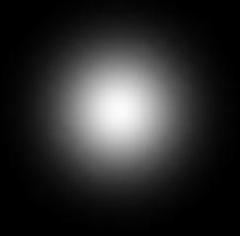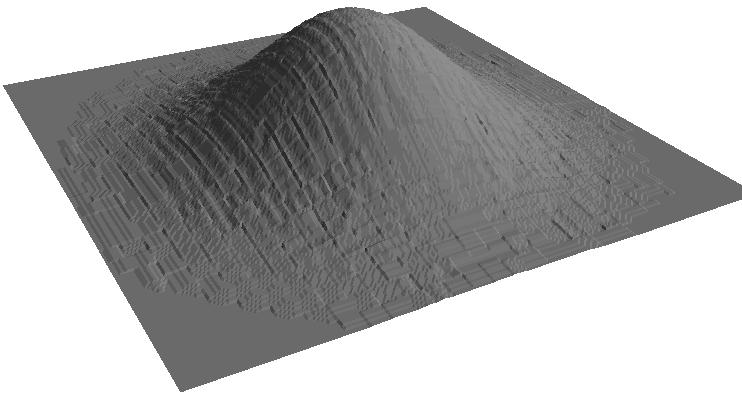meshpro bunny.off gaussian.off -image gaussian.jpg

## Expected Point Earnings

Bold means required
 Operation Points Random Noise 1 Fun 1 Scale 1 Rotate 1 Inflate 1 Deform 3 Smooth 2 Sharpen 2 Subdivide 2 Compute Normals 2 Surface of Revolution 2 Surface Sweep 2 Fractalize 2 Fill Holes 2 Create Mesh from Image 2 Art Contest Submission 1 Total 27